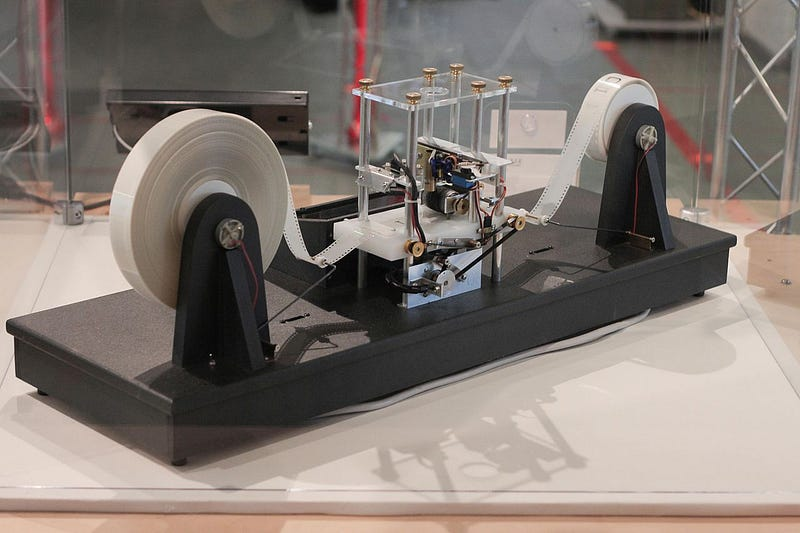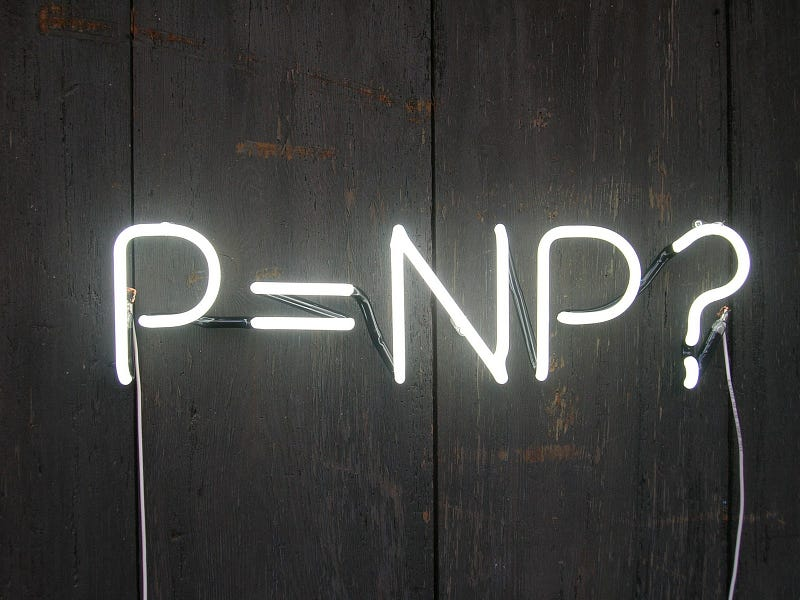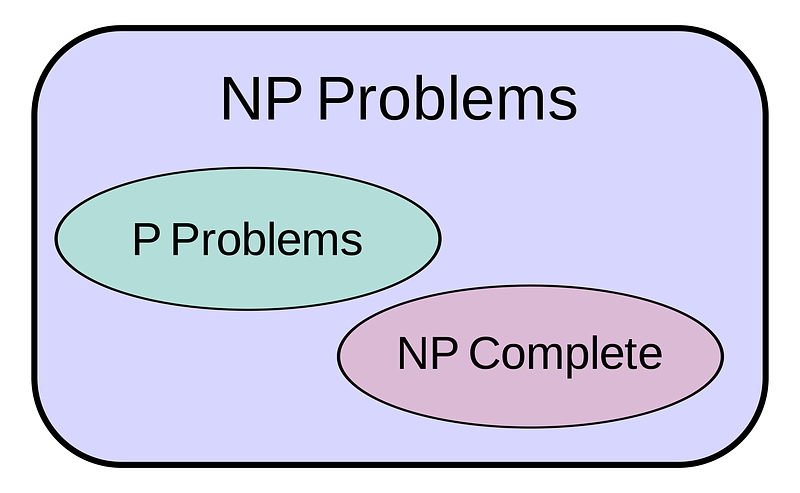Search

# Computability and problem-solving: part one.

You can also read this story on medium.

The divide between the theoretical computer scientist and the software engineer has never been larger. The rapid expansion of this valley has left a vacuum in many engineers that if neatly filled with theoretical concepts and stitched together with intuitive links can prove to be a powerful utility for advanced problem-solving and design alike.https://www.wikiwand.com/simple/Turing_machine

This series of essays attempts to explore one aspect of theoretical computer science through a conceptual lens designed to bring forth pragmatic applications of these highly theoretical concepts that can be interpreted as additional dimensions in an engineer’s space of problem-solving.

Together, we will build from the ground up our own little intuitive interpretation of a portion of computability theory and apply that intuition in a brief study of a famously purely functional programming language: Haskell.

Here is an overview of what this series plans to touch on:

1. An introduction to problems.

2. An introduction to computability theory.

3. Turing machines and nondeterminism.

4. Lambda calculus.

5. The Game of Life.

7. The fabric that holds all of this together.

By the end of this series, I hope the reader comes out with a new-found admiration for the applicability of such theoretical concepts to the software engineer’s daily problem-solving life.

To be clear, this series is not meant to be some kind of resource in formal theoretical computer science: in fact, quite the contrary. It is meant to shed light on fundamental concepts in an inherently informal way as an effort to build subject intuition. Throughout the series, I will be linking additional resources (mostly in the form of notes, papers, and Wikipedia articles) to afford anyone particularly interested in a certain topic the opportunity to dive deeper into it; however, none of what I link to is required to follow along.

There won’t be any fancy symbols or rigorous proofs, but if you’re interested in exploring the formalities of computability and proof theory, a great place to start is definitely Stephen Cook’s Computability and Logic course at the University of Toronto. Stephen Cook, in his seminal 1971 paper The Complexity of Theorem-Proving Procedures, famously formulated the P versus NP problem which is, not very coincidentally, where we will begin.https://jeremykun.com/2012/02/23/p-vs-np-a-primer-and-a-proof-written-in-racket/

## The P versus NP problem.

The P versus NP problem is a classic unsolved problem in theoretical computer science (it is, in fact, one of the Millenium Prize Problems); furthermore, its relative popularity in the computer science landscape makes it a prime location to kick of this discussion of theory. We will use the understanding behind this famous problem as an initial target towards which we build our intuitive interpretation of computability theory.

The P versus NP problem asks whether every problem whose solution can be quickly verified can also be solved quickly.

This deceptively simple statement takes a good amount of complex mathematical machinery to rigorously define, but in the spirit of this series, we will tackle it on a conceptual basis, beginning with the idea of “solving a problem”.

## What is a “problem”?

### First attempt at a definition.

The theoretical concept of a problem in computer science might come off as a bit too optimistic at first glance:

A problem is anything that can be solved

This unsatisfying definition might feel somewhat cheaty, but I promise it segues into much more fulfilling ideas.

It would be surprising if this definition did not raise some eyebrows or bring up a few concerns. We know of “problems” that are inherently unsolvable by any algorithm, is this definition exiling these “problems” from being problems at all? No, that would defeat the purpose. A key element to notice here is that there are problems that are unsolvable by any algorithm, but not any thing. More on that in a bit.

We will talk a lot more about what it means to be an algorithm later in this series, but for now, let’s think about what this thing that can solve a problem that no algorithm can solve might look like.

### Machines.https://en.wikipedia.org/wiki/Black_box

From here on out in the series, we will be using the term machine quite a lot:

A machine is a construct that takes in an input and might produce an output in finite amount of time.

Simply put, a machine is something that, by some unknown or even impossible process, might produce an output when given some input. We say might here because some machines do not produce an output for every possible input. For example, a machine that adds two numbers correctly probably should not output anything if I give it the inputs of “Hello” and “World” since they are not numbers.

Remember that a machine can be anything, it doesn’t need to be implemented or designed: if I say to construct a machine X that adds two numbers correctly then that machine now exists, regardless of how this addition actually happens.

### Second attempt at a definition.

By the arcane magic that is our imagination, let us conjure up a black box machine (i.e. a thing that takes in an input and might produce an output) of sorts capable of answering any question we might have, given that the inputted question is well-defined enough to have a set of valid answers. We call machines like these oracles; in this case, we will call this machine the all-knowing oracle machine.

With this idea in mind, we can come up with a slightly more satisfying definition of a problem:

A problem is a question that has a (well-defined, but possibly empty) set of answers, potentially (but not necessarily) dependent on a (possibly empty) set of inputs.

We silently introduced this concept of “inputs” in the most recent definition, this is required to formalize the idea of a problem potentially being a collection of questions rather than a singular question. For example, the question of “what is x plus y?” warrants an answer of “it depends on x and y”; however, coupled with inputs such as “x is 10” and “y is 2” we get a well-defined answer of 12. Hence we can use our all-knowing oracle machine to “solve” this problem of “what is x plus y?” by taking in as input the values of “x” and “y” and passing in the constructed question of “what is x plus y?” with the values replaced to our all-knowing oracle machine and then forwarding the answer it gives as our output. In a sense, we are using the fact that our all-knowing oracle machine can solve all instances of this problem to solve the problem as a whole.

The set of problem instances is precisely the set of valid inputs to our all-knowing oracle machine. Another definition of a problem instance is a question that has a well-defined set of solutions.

### Third attempt at a definition.

With these concepts in mind, we can come up with our final (and in my opinion, very pretty) definition of what a problem is and consequently what solving a problem means:

A problem is a non-empty (possibly infinite) set of input-answers tuples.
A machine solves a problem if it produces a valid answer to each instance of this problem given the associated input.

Under this definition, our all-knowing oracle machine does not, without modification, solve the “what is x plus y?”; in fact, it is incompatible with solving this problem since the all-knowing oracle machine takes in one input (a problem instance) while this problem requires the machine to accept two inputs (x and y). We demonstrated how the all-knowing oracle machine could be used to solve this problem a few paragraphs previous.

### Some examples.

To solidify the formal concept of a “problem” we will run through a few examples.

“Does God exist?” — supposing that all associated terms are well-defined (i.e. what “God” is and what constitutes “existence”), this is a nullary decision problem. “Nullary” meaning that it has zero inputs and “decision” meaning that the output is either True or False. In the case where some term is not properly defined, such as “existence” this ceases to be a problem unless you relax the problem to be a unary (taking in one input) decision problem where the input is the proper definition of “existence”.

“What is 1 plus 2?” — supposing that we are operating under the standard rules of mathematics with the naturals, this is a nullary function problem. Where “function” means that the output can be any single element (and hence decision problems are a special case of function problems).

“What is x plus y?” — again supposing the standard rules of mathematics with the naturals, this is a binary function problem. “Binary” meaning that the problem has two inputs.

“Is P=NP?” — this one should seem a bit familiar. The P versus NP problem is, perhaps unsurprisingly, a problem of its own. It is a nullary decision problem.

The P versus NP problem asks whether every problem whose solution can be quickly verified can also be solved quickly.

The problem statement itself refers to other problems, which is fine, it is a meta-problem of sorts. In fact, the P versus NP problem does apply to itself in a sense.

### Thoughts on the formality of problems.

A recurring theme that has somewhat been swept under the rug in this section has been the idea of “context” or “definition”. In all of these problems, we are assuming that the semantic meaning of these sentences is precisely defined, with no room for ambiguity. However, we know for a fact that the English language can somewhat be ambiguous and that the “contextual definitions” of many terms can vary.

To remedy this issue, we always formally define problems in a logical system such as propositional logic or first-order logic with an associated meaning to each symbol, in which there is zero room for ambiguity. For simplicity and for the sake of staying away from a tiring mathematical burden, throughout this series we will be presenting problems and statements in English with as much ambiguity explained away as possible. When not stated, assume the regular or standard definition of the word or symbol.

### Decision problems.

Solving problems form the basis of theoretical computer science. There are many different classes of computational problems; however, I would argue the most important would be the decision problem.

As we mentioned previously, a decision problem is one where the answer is always either True or False (equivalently Yes or No and 1 or 0). These problems are the simplest computation problems to structure, reason about, and sometimes define; although the concepts introduced on behalf of them extend easily and quickly to all other decision problems.

For the rest of this series, we will mostly focus on decision problems, but it might be a good test of understanding to think about how the concepts introduced relative to decision problems can be reframed to other classes of problems such as function problems.

### Constructing machines to solve problems.

By utilizing the existence of our all-knowing oracle machine, we can construct machines that can solve arbitrary problems. This concept of utilizing machines as a subprocedure in the construction of other machines should seem pretty familiar: we do it any time we call a function in our favorite programming language.

Since a machine, by definition, can operate based on any underlying process, it definitely makes sense to use the all-knowing oracle machine as the ultimate “solver” and just utilizing the rest of our constructed machine to correctly format the input and output. We have already done this in the section about the second attempt at a definition of “problem”.

Using our all-knowing oracle machine as a subroutine might raise the question of “why bother”? If we can already just imagine machines to bring them into existence, why not just imagine a new machine for each problem we want to solve. That is a very fair point; however, being able to do this breaks down once we start to talk about machines that we can implement.

## Back to the bigger picture.

We just spent a whole lot of time building up the concept of a problem and solidifying it in our intuitive framework, along with what it means for a machine to solve a problem. We talked about oracle machines and how these magic boxes can solve any problem instance we throw at them, and we talked about how we might use these oracle machines to solve arbitrary problems. What we haven’t talked about, though, is how we would go about constructing one of these oracle machines to run on our computers in order to solve all of our problems.

In fact, there are a whole lot of things we can’t do on our computers; even more disappointingly, there are problems that no process that we can simulate can solve. That is, there are some problems that are only solved by processes that we cannot possibly compute, however powerful our computers might be.

Before we can proceed, we need to talk about what it means to “compute” things; and to do that we need to start diving into computability theory.

We will begin to explore computability theory (and more generally the theory of computation) in the next essay of this series. For now, let’s touch on P versus NP one last time.

## The P versus NP problem, again.https://en.wikipedia.org/wiki/P_versus_NP_problem
The P versus NP problem asks whether every problem whose solution can be quickly verified can also be solved quickly.

The skeptical of you might argue that with this statement of the problem and our previous definitions of “problem” and “solution”, the answer is trivial: yes, since we can construct an oracle machine that will solve the problem in one step (so pretty quickly).

Did we just solve the P versus NP problem? Well, no. When we say solving or verifying quickly, in this context, we mean the existence of a computable algorithm that can solve the problem in a polynomial number of steps. Again, we will get to what we mean by a “computable algorithm” in the next essay of this series; however, a polynomial number of steps here just means that the number of operations that our machine does grows at most polynomially with the size of the input to the machine.

This, however, raises the question of what constitutes an operation: what if I just define an “operation” to be the operation of solving my problem, then I am solving it trivially in one operation, am I not? Well, yes and no. What an operation constitutes is actually well-defined, but the explanation of that will also come once we start discussing computability theory.

## Wrapping up.

We went over a lot of stuff this essay and we have laid the foundation for the rest of this series. Throughout the series, we will be talking about problems a lot, so take some time to make sure that the intuitive idea of a problem in the context of theoretical computer science has rested in your mind.

We still need to clear a few things up in the next essay. What does it mean to be an algorithm, and similarly what does it mean to compute or be computable? What is the formal definition of an operation (this will probably come in the essay about Turing machines)? How does computability theory play into this?

There is a long way to go until we can start to frame our problem-solving around these theoretical concepts; however, I hope that by now the reader has gained some sort of appreciation for the utility that formalizing common concepts might afford in problem-solving, even though we still have not talked about how we can approach solving problems through this conceptual lens.

Thanks for reading, feel free to reach out to me through any of the contact methods available on my website in the header if you have any questions or concerns. Follow me on Twitter to read about other random dumb stuff I say.